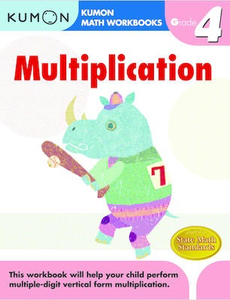# Grade 4 Multiplication

• \$8.95

Multiplication, Grade 4 is in the "Kumon Math Workbooks: Calculation Skills" series designed for grades 1-6. This workbook will introduce students to subtracting one-digit numbers from one-and-two digit numbers. Exercises include drill multiplication problems as well as word problem exercises where students fill in the missing numbers by using multiplication patterns. Activities also emphasize vertical form multiplication; three-digit times three-digit multiplication; the commutative property of multiplication; and patterns & sequencing. Pages provide the number of points to assign per problem, and there's room at the top to write in how many problems students got right alongside their name and the date.